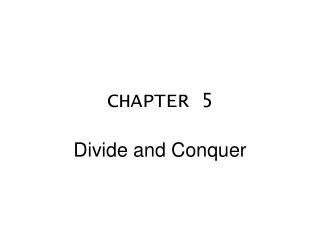Download PresentationCHAPTER 5

# CHAPTER 5 - PowerPoint PPT PresentationDownload Presentation## CHAPTER 5

- - - - - - - - - - - - - - - - - - - - - - - - - - - E N D - - - - - - - - - - - - - - - - - - - - - - - - - - -
##### Presentation Transcript

1. CHAPTER 5 Divide and Conquer

2. Algorithm 5.1.4 Tiling a Deficient Board with Trominoes This algorithm constructs a tiling by trominoes of a deficient n×n board where n is a power of 2.

3. Input Parameters: n, a power of 2 (the board size); the location L of the missing square Output Parameters: None tile(n,L) { if (n == 2) { // the board is a right tromino T tile with T return } divide the board into four n/2 × n/2 subboards place one tromino as in Figure 5.1.4(b) // each of the 1 × 1 squares in this tromino // is considered as missing let m1,m2,m3,m4 be the locations of the missing squares tile(n/2,m1) tile(n/2,m2) tile(n/2,m3) tile(n/2,m4) }

4. Algorithm 5.2.2 Merge This algorithm receives as input indexes i, m, and j, and an array a, where a[i], ... , a[m] and a[m +1], ... , a[j] are each sorted in nondecreasing order. These two nondecreasing subarrays are merged into a single nondecreasing array.

5. Input Parameters: a,i,m,j Output Parameter: a merge(a,i,m,j) { p = i // index in a[i], ... , a[m] q = m + 1 // index in a[m + 1], ... , a[j] r = i // index in a local array c while (p ≤ m && q ≤ j) { // copy smaller value to c if (a[p] ≤ a[q]) { c[r] = a[p] p = p + 1 } else { c[r] = a[q] q = q + 1 } r = r + 1 } ...

6. ... // copy remainder, if any, of first subarray to c while (p ≤ m) { c[r] = a[p] p = p + 1 r = r + 1 } // copy remainder, if any, of second subarray to c while (q ≤ j) { c[r] = a[q] q = q + 1 r = r + 1 } // copy c back to a for r = i to j a[r] = c[r] }

7. Algorithm 5.2.3 Mergesort This algorithm sorts the array a[i], ... , a[j] in nondecreasing order. It uses the merge algorithm (Algorithm 5.2.2). Input Parameters: a,i,j Output Parameter: a mergesort(a,i,j) { // if only one element, just return if (i == j) return // divide a into two nearly equal parts m = (i + j)/2 // sort each half mergesort(a,i,m) mergesort(a,m + 1,j) // merge the two sorted halves merge(a,i,m,j) }

8. Algorithm 5.3.2 Finding the Distance Between a Closest Pair of Points This algorithm finds the distance between a closest pair of points. The input is an array p, ... , p[n] of n = 2 points. If p is a point, p.x is the x- coordinate of p, and p.y is the y-coordinate of p. The function merge is Algorithm 5.2.2 and mergesort is Algorithm 5.2.3. The function merge uses as the key the y-coordinate of the point. The function mergesort uses as the key either the x- or y-coordinate of the point; the comments indicate which. The function dist(p,q) returns the Euclidean distance between points p and q.

9. Input Parameters: p Output Parameter: None closest_pair(p) { n = p.last mergesort(p,1,n) // sort by x-coordinate return rec_cl_pair(p,1,n) } // rec_cl_pair assumes that input is sorted by x-coordinate. // At termination, the input is sorted by y-coordinate. rec_cl_pair(p,i,j) { if (j - i < 3) { mergesort(p,i,j) // sort by y-coordinate // find the distance delta between a closest pair delta = dist(p[i],p[i + 1]) if (j - i == 1) // two points return delta // three points if (dist(p[i + 1],p[i + 2]) < delta) delta = dist(p[i + 1],p[i + 2]) if (dist(p[i],p[i + 2]) < delta) delta = dist(p[i],p[i + 2]) return delta } ...

10. ... k = (i + j)/ 2 l = p[k].x deltaL = rec_cl_pair(p,i,k) deltaR = rec_cl_pair(p,k + 1,j) delta = min(deltaL,deltaR) // p[i], ... , p[k] is now sorted by y-coordinate, and // p[k + 1], ... , p[j] is now sorted by y-coordinate. merge(p,i,k,j) // p[i], ... , p[j] is now sorted by y-coordinate. // store points in the vertical strip in v. t = 0 for k = i to j if (p[k].x > l - delta && p[k].x < l + delta) { t = t + 1 v[t] = p[k] } // look for closer pairs in the strip by comparing // each point in the strip to the next 7 points. for k = 1 to t - 1 for s = k + 1 to min(t,k + 7) delta = min(delta,dist(v[k],v[s])) return delta }

11. Algorithm 5.4.1 Matrix Product This algorithm computes the product C of the n×n matrices A and B directly from the definition of the matrix product. Input Parameters: A,B Output Parameter: C matrix_product(A,B,C) { n = A.last for i = 1 to n for j = 1 to n { C[i][j] = 0 for k = 1 to n C[i][j] = C[i][j] + A[i][k] * B[k][j] } }